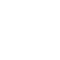# Today we saw the introduction of the LAMBDA feature in Excel by Microsoft, which will revolutionize the formulation of this program by providing the ability to create formulas as a function in Excel itself.

Today, Microsoft has introduced a new feature for the Excel application that revolutionizes formulation in Excel.

As you know, writing formulas in Excel is very important and useful, but to date, it was not possible to write formulas as a function (which can be reused),

using the language Excel itself, and the user had to use languages ​​such as Use JavaScript.

Now, thanks to the LAMBDA feature, you can write the function you want using the Excel formula. On the other hand, with this feature, one function can call another function.

## Functions that can be reused

With this feature you can create any function in Excel, put it in a LAMBDA function, give it a name, and then use it anywhere in the Excel sheet.

## Recursive functions

Although reusable Custom Functions are in themselves a good reason to take advantage of the new Excel feature, a positive feature that LAMBDA adds to Excel is the recursive functions.

For example,

if you have created a LAMBDA called MYFUNC, you can call this function within itself. It is interesting to know that before LAMBDA in Excel,

this feature was only possible with scripts such as JavaScript, VBA, etc.

## Definition of a LAMBDA

In the image below, you can see how to define a LAMBDA, which consists of two parts: parameters and calculation.

In the Parameters section, you will enter the value you want to send as input to the function (such as number, string, etc.).

And in the calculation section, you must enter the formula that you want to run.